# Baonza equation of state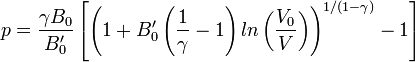$p=\frac{\gamma B_0}{B_0'}\left[\left(1+B_0'\left(\frac{1}{\gamma}-1\right)ln\left(\frac{V_0}{V}\right)\right)^{1/(1-\gamma)}-1\right]$
where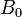$B_0$ is the isothermal bulk modulus,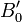$B_0'$ is the pressure derivative of the bulk modulus and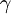$\gamma$ relates the bulk modulus and its pressure derivative via: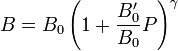$B=B_0\left(1+\frac{B_0'}{B_0}P\right)^{\gamma}$